### ImageProcessing.Compute.Color.BrightnessContrast

This node allows changing the brightness and contrast of an image. A brightness offset is selected via the Brightness input slot. A contrast offset can be specified via the Contrast input slot. Both in the range [-100, 100].

The effect of brightness and contrast change can be best observed on a gray scale gradient image. A negative brightness offset condenses all color values towards black. A positive brightness offset condenses all values towards white.

 Brightness offset: -100 -75 -50 -25 0 25 50 75 100 Result: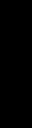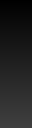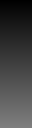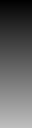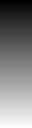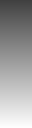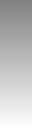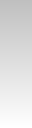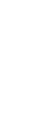A negative contrast offset moves all color values more towards medium gray. A positive contrast offset spreads the color values away from medium gray.

 Contrast offset: -100 -75 -50 -25 0 25 50 75 100 Result: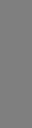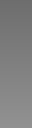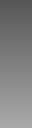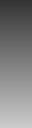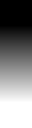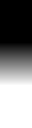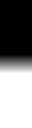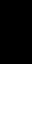#### Brightness

To understand what happens exactly during a brightness change, the diagrams below show the mapping curves between grayscale values.In the left diagram, the brightness is decreased. For a brightness offset of 0 nothing should change and the mapping curve is a straight line with a gradient of 1. For a brightness offset of -50 the gradient is reduced to 0.5. And for a brightness offset of -100 the gradient is 0. Mathematically, this is can be expressed as

$\begin{pmatrix}R_{\mathrm{out}}\\G_{\mathrm{out}}\\B_{\mathrm{out}}\end{pmatrix} = \left(1.0 + \frac{b}{100}\right) \, \begin{pmatrix}R_{\mathrm{in}}\\G_{\mathrm{in}}\\B_{\mathrm{in}}\end{pmatrix} \,\,:\,\, b \le 0 \quad,$
where $R$, $G$, $B$ are RGB color values in the range [0.0, 1.0] and $b$ is the selected brightness offset in the range [-100, 0].

In the right diagram, the brightness is increased. Again, for a brightness offset of 0 the mapping curve is a straght line with a gradient of 1. For a brightness offset of +50 the mapping curve has a gradient of 0.5 and a y-intercept of 0.5. For a brightness offset of +100 the gradient is 0 and the y-offset is 1.0. This mapping is given by the equation:

$\begin{pmatrix}R_{\mathrm{out}}\\G_{\mathrm{out}}\\B_{\mathrm{out}}\end{pmatrix} = \left(1.0 - \frac{b}{100}\right) \, \begin{pmatrix}R_{\mathrm{in}}\\G_{\mathrm{in}}\\B_{\mathrm{in}}\end{pmatrix} + \begin{pmatrix} \frac{b}{100} \\ \frac{b}{100}\\ \frac{b}{100}\end{pmatrix}\,\,:\,\, b > 0 \quad,$
where the brightness offset $b$ is now in range [0, 100].

#### Contrast

The diagrams below show the mapping curves between grayscale values for a decrease (left) and increase (right) of contrast.In the left diagram, the contrast is decreased. For a contrast offset of 0 the mapping curve is a straight line with a gradient of 1. For other contrast offsets the mapping curve rotates around medium gray. Thereby the gradient of the line becomes smaller for larger negative contrast offsets until the gradient is zero for a contrast offset of -100.

In the right diagram, the contrast is increased. Again, the line rotates around medium gray. The gradient of the line becomes larger for larger positive contrast offsets until the line is almost vertical for a contrast offset of +100. However, typically it is sufficient to have a certain maximal gradient. Asuming 8-bit color depth, a maximal gradient of 256 is chosen for a contrast offset of +100.

Mathematically the mapping can be expressed as

$\begin{pmatrix}R_{\mathrm{out}}\\G_{\mathrm{out}}\\B_{\mathrm{out}}\end{pmatrix} = g \, \begin{pmatrix}R_{\mathrm{in}}-0.5\\G_{\mathrm{in}}-0.5\\B_{\mathrm{in}}-0.5\end{pmatrix} + \begin{pmatrix} 0.5 \\ 0.5\\ 0.5\end{pmatrix} \quad,$
where $R$, $G$, $B$ are RGB color values in the range [0.0, 1.0] and $g$ is the gradient of the mapping curve.

The gradient of the mapping curve $g$ can be computed from the contrast offset $c$ considering the following three constraints: $c=-100 \mapsto g=0$,   $c=0 \mapsto g=1$,   $c=\,\,100 \mapsto g=256$.

A suitable function that fulfills these constraints is:

$g = \frac{c + 100}{100- \frac{127 \,c}{128}} \quad.$

By combining both equations, we get:

$\begin{pmatrix}R_{\mathrm{out}}\\G_{\mathrm{out}}\\B_{\mathrm{out}}\end{pmatrix} = \frac{c + 100}{100- \frac{127 \,c}{128}} \, \begin{pmatrix}R_{\mathrm{in}}-0.5\\G_{\mathrm{in}}-0.5\\B_{\mathrm{in}}-0.5\end{pmatrix} + \begin{pmatrix} 0.5 \\ 0.5\\ 0.5\end{pmatrix}\quad,$
where $R$, $G$, $B$ are RGB color values in the range [0.0, 1.0] and $b$ is the contrast offset in range [-100, 100].